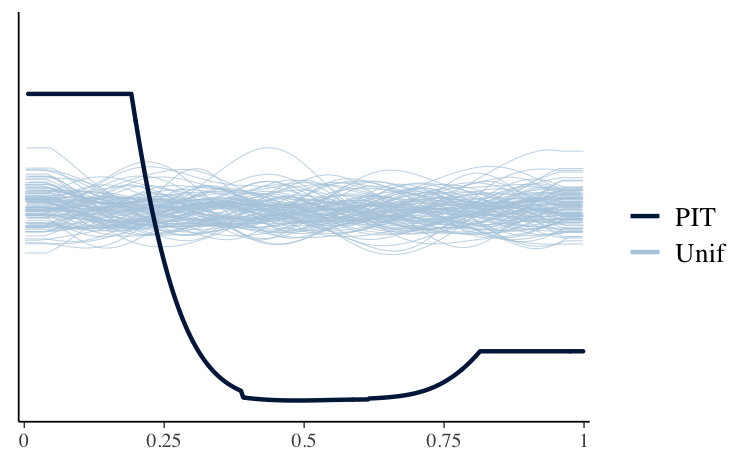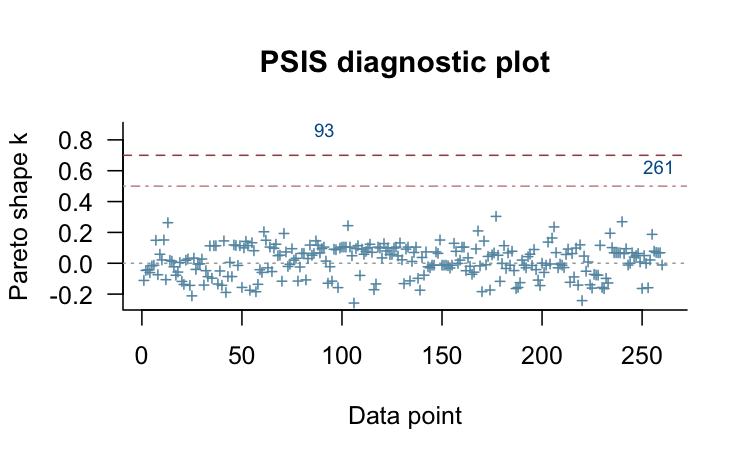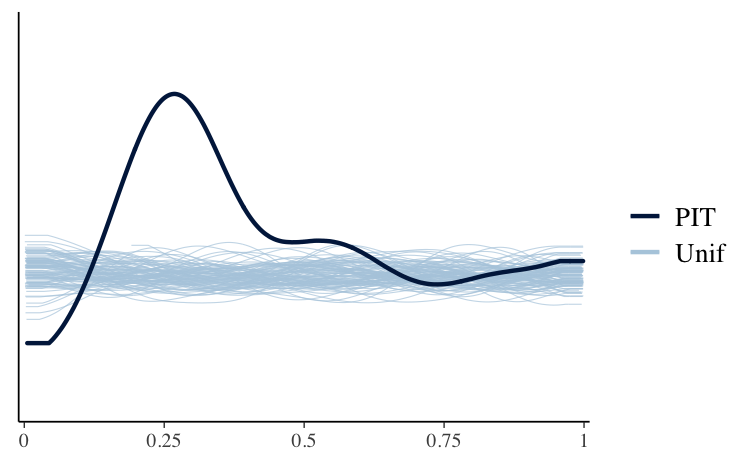# Introduction

This vignette demonstrates how to use the loo package to carry out Pareto smoothed importance-sampling leave-one-out cross-validation (PSIS-LOO) for purposes of model checking and model comparison.

In this vignette we can’t provide all necessary background information on PSIS-LOO and its diagnostics (Pareto $$k$$ and effective sample size), so we encourage readers to refer to the following papers for more details:

• Vehtari, A., Gelman, A., and Gabry, J. (2017). Practical Bayesian model evaluation using leave-one-out cross-validation and WAIC. Statistics and Computing. 27(5), 1413–1432. :10.1007/s11222-016-9696-4. Links: published | arXiv preprint.

• Vehtari, A., Simpson, D., Gelman, A., Yao, Y., and Gabry, J. (2019). Pareto smoothed importance sampling. arXiv preprint arXiv:1507.04544.

# Setup

In addition to the loo package, we’ll also be using rstanarm and bayesplot:

library("rstanarm")
library("bayesplot")
library("loo")

# Example: Poisson vs negative binomial for the roaches dataset

## Background and model fitting

The Poisson and negative binomial regression models used below in our example, as well as the stan_glm function used to fit the models, are covered in more depth in the rstanarm vignette Estimating Generalized Linear Models for Count Data with rstanarm. In the rest of this vignette we will assume the reader is already familiar with these kinds of models.

### Roaches data

The example data we’ll use comes from Chapter 8.3 of Gelman and Hill (2007). We want to make inferences about the efficacy of a certain pest management system at reducing the number of roaches in urban apartments. Here is how Gelman and Hill describe the experiment and data (pg. 161):

the treatment and control were applied to 160 and 104 apartments, respectively, and the outcome measurement $$y_i$$ in each apartment $$i$$ was the number of roaches caught in a set of traps. Different apartments had traps for different numbers of days

In addition to an intercept, the regression predictors for the model are roach1, the pre-treatment number of roaches (rescaled above to be in units of hundreds), the treatment indicator treatment, and a variable indicating whether the apartment is in a building restricted to elderly residents senior. Because the number of days for which the roach traps were used is not the same for all apartments in the sample, we use the offset argument to specify that log(exposure2) should be added to the linear predictor.

# the 'roaches' data frame is included with the rstanarm package
data(roaches)
str(roaches)
'data.frame':   262 obs. of  5 variables:
$y : int 153 127 7 7 0 0 73 24 2 2 ...$ roach1   : num  308 331.25 1.67 3 2 ...
$treatment: int 1 1 1 1 1 1 1 1 0 0 ...$ senior   : int  0 0 0 0 0 0 0 0 0 0 ...
yrep = yrep,
lw = weights(loo1$psis_object) ) NOTE: The kernel density estimate assumes continuous observations and is not optimal for discrete observations.The excessive number of values close to 0 indicates that the model is under-dispersed compared to the data, and we should consider a model that allows for greater dispersion. ## Try alternative model with more flexibility Here we will try negative binomial regression, which is commonly used for overdispersed count data. Unlike the Poisson distribution, the negative binomial distribution allows the conditional mean and variance of $$y$$ to differ. fit2 <- update(fit1, family = neg_binomial_2) loo2 <- loo(fit2, save_psis = TRUE, cores = 2) Warning: Found 1 observation(s) with a pareto_k > 0.7. We recommend calling 'loo' again with argument 'k_threshold = 0.7' in order to calculate the ELPD without the assumption that these observations are negligible. This will refit the model 1 times to compute the ELPDs for the problematic observations directly. print(loo2)  Computed from 4000 by 262 log-likelihood matrix Estimate SE elpd_loo -895.6 37.8 p_loo 6.7 2.7 looic 1791.3 75.5 ------ Monte Carlo SE of elpd_loo is NA. Pareto k diagnostic values: Count Pct. Min. n_eff (-Inf, 0.5] (good) 260 99.2% 2615 (0.5, 0.7] (ok) 1 0.4% 377 (0.7, 1] (bad) 1 0.4% 28 (1, Inf) (very bad) 0 0.0% <NA> See help('pareto-k-diagnostic') for details. plot(loo2, label_points = TRUE)Using the label_points argument will label any $$k$$ values larger than 0.7 with the index of the corresponding data point. These high values are often the result of model misspecification and frequently correspond to data points that would be considered outliers’’ in the data and surprising according to the model Gabry et al (2019). Unfortunately, while large $$k$$ values are a useful indicator of model misspecification, small $$k$$ values are not a guarantee that a model is well-specified. If there are a small number of problematic $$k$$ values then we can use a feature in rstanarm that lets us refit the model once for each of these problematic observations. Each time the model is refit, one of the observations with a high $$k$$ value is omitted and the LOO calculations are performed exactly for that observation. The results are then recombined with the approximate LOO calculations already carried out for the observations without problematic $$k$$ values: if (any(pareto_k_values(loo2) > 0.7)) { loo2 <- loo(fit2, save_psis = TRUE, k_threshold = 0.7) } 1 problematic observation(s) found. Model will be refit 1 times.  Fitting model 1 out of 1 (leaving out observation 93) print(loo2)  Computed from 4000 by 262 log-likelihood matrix Estimate SE elpd_loo -895.5 37.7 p_loo 6.6 2.6 looic 1791.1 75.4 ------ Monte Carlo SE of elpd_loo is 0.2. Pareto k diagnostic values: Count Pct. Min. n_eff (-Inf, 0.5] (good) 260 99.6% 2615 (0.5, 0.7] (ok) 1 0.4% 377 (0.7, 1] (bad) 0 0.0% <NA> (1, Inf) (very bad) 0 0.0% <NA> All Pareto k estimates are ok (k < 0.7). See help('pareto-k-diagnostic') for details. In the print output we can see that the Monte Carlo SE is small compared to the other uncertainties. On the other hand, p_loo is about 7 and still a bit higher than the total number of parameters in the model. This indicates that there is almost certainly still some degree of model misspecification, but this is much better than the p_loo estimate for the Poisson model. For further model checking we again examine the LOO-PIT values. yrep <- posterior_predict(fit2) ppc_loo_pit_overlay(roaches$y, yrep, lw = weights(loo2\$psis_object))
NOTE: The kernel density estimate assumes continuous observations and is not optimal for discrete observations.The plot for the negative binomial model looks better than the Poisson plot, but we still see that this model is not capturing all of the essential features in the data.

## Comparing the models on expected log predictive density

We can use the loo_compare function to compare our two models on expected log predictive density (ELPD) for new data:

loo_compare(loo1, loo2)
     elpd_diff se_diff
fit2     0.0       0.0
fit1 -5352.2     709.2

The difference in ELPD is much larger than several times the estimated standard error of the difference again indicating that the negative-binomial model is expected to have better predictive performance than the Poisson model. However, according to the LOO-PIT checks there is still some misspecification, and a reasonable guess is that a hurdle or zero-inflated model would be an improvement (we leave that for another case study).